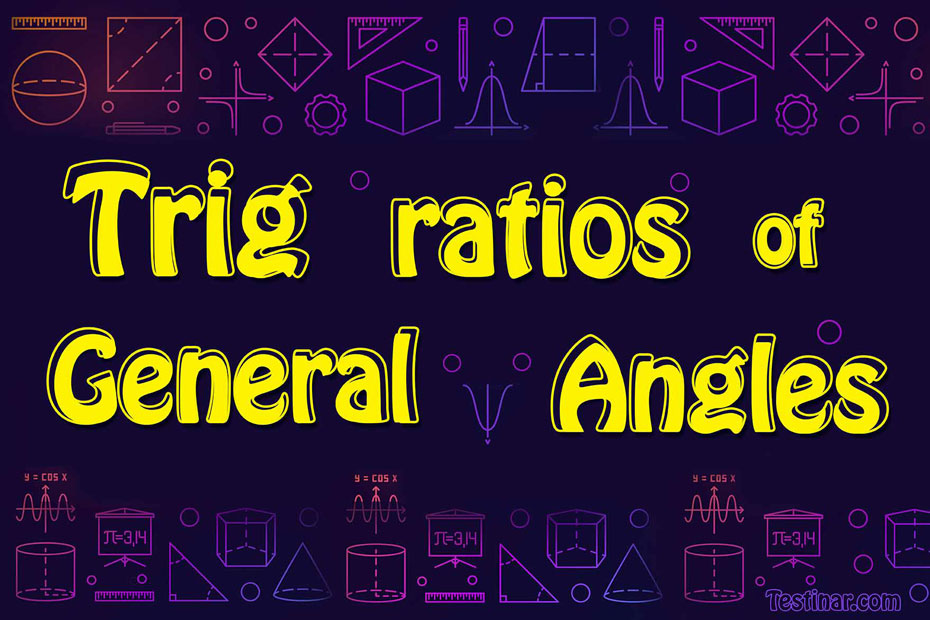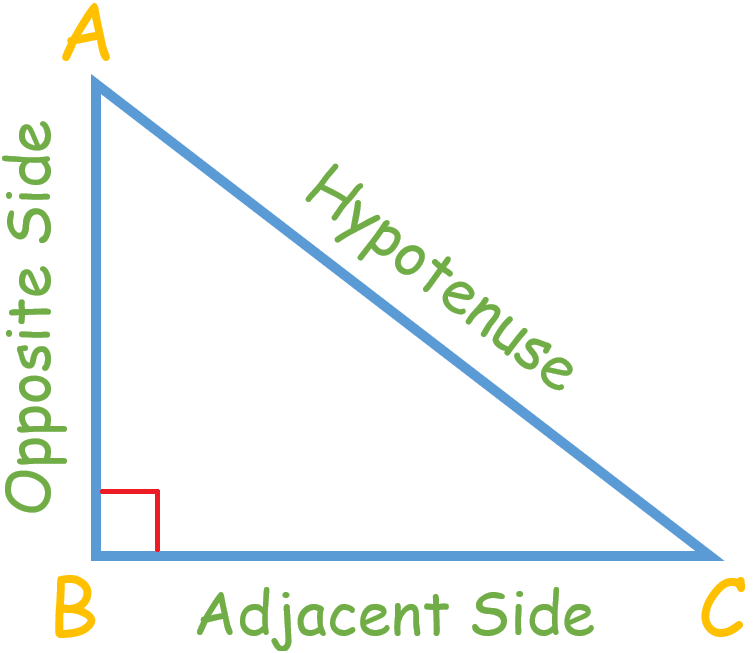## How to Find Trigonometric Ratios of General Angles

### Trig ratios of General Angles

Sine, Cosine, Tangent, Cotangent, Secant, and Cosecant are trigonometric ratios. For these trigonometric ratios, the standard angles are $$0, \ 30, \ 45, \ 60,$$ and $$90$$ degrees. These angles can also be shown using radians, such as $$0, \ \frac{π}{6}, \ \frac{π}{4}, \ \frac{π}{3},$$ and $$\frac{π}{2}$$ . In trigonometry, these angles are most regularly and frequently used. To solve many problems, you need to know the values of these trigonometry angles.

Here are the trigonometry ratios for a particular $$θ$$ angle:

 $$Sin \ θ$$ $$\frac{Opposite \ Side \ to \ θ}{Hypotenuse}$$ $$Cos \ θ$$ $$\frac{Adjacent \ Side \ to \ θ}{Hypotenuse}$$ $$Tan \ θ$$ $$\frac{Opposite \ Side \ to \ θ}{Adjacent \ Side \ to \ θ}$$ OR $$\frac{Sin \ θ}{Cos \ θ}$$ $$Cot \ θ$$ $$\frac{Adjacent \ Side \ to \ θ}{Opposite \ Side \ to \ θ}$$ OR $$\frac{Cos \ θ}{Sin \ θ} \ = \ \frac{1}{tan \ θ}$$ $$Sec \ θ$$ $$\frac{Hypotenuse}{Adjacent \ Side \ to \ θ}$$ OR $$\frac{1}{cos \ θ}$$ $$Cosec \ θ$$ $$\frac{Hypotenuse}{Opposite \ Side \ to \ θ}$$ OR $$\frac{1}{sin \ θ}$$

### Finding Trigonometric Ratios

Consider this right triangle:The trigonometric ratios of $$∠ \ C$$ are:

• Sine: The ratio of an angle's perpendicular (opposite) side to its hypotenuse is known as its sine.
• Cosine: The ratio of the side adjacent to the angle to the hypotenuse is known as the cosine of an angle.
• Tangent: The tangent of an angle is the ratio between the side opposite the angle and the side adjacent to it.
• cotangent: tangent's multiplicative inverse is cotangent.
• Cosecant: Sine's multiplicative inverse is cosecant.
• Secant: cosine's multiplicative inverse is Secant.

In the order they are listed, the above ratios are written as $$sin, \ cos, \ tan, \ cosec, \ sec,$$ and $$tan$$. So, in the case of $$Δ \ ABC$$, the ratios are:

$$Sin \ C \ = \ \frac{Opposite \ Side \ to \ ∠ \ C}{Hypotenuse} \ = \ \frac{AB}{AC}$$

$$Cos \ C \ = \ \frac{Adjacent \ Side \ to \ ∠ \ C}{Hypotenuse} \ = \ \frac{BC}{AC}$$

$$tan \ C \ = \ \frac{Opposite \ Side \ to \ ∠ \ C}{Adjacent \ Side \ to \ ∠ \ C} \ = \ \frac{AB}{BC} \ = \ \frac{Sin \ C}{Cos \ C}$$

$$Cot \ C \ = \ \frac{Adjacent \ Side \ to \ ∠ \ C}{Opposite \ Side \ to \ ∠ \ C} \ = \ \frac{BC}{AB} \ = \ \frac{1}{tan \ C}$$

$$Cosec \ C \ = \ \frac{Hypotenuse}{Opposite \ Side \ to \ ∠ \ C} \ = \ \frac{AC}{AB} \ = \ \frac{1}{Sin \ C}$$

$$Sec \ C \ = \ \frac{Hypotenuse}{Adjacent \ Side \ to \ ∠ \ C} \ = \ \frac{AC}{BC} \ = \ \frac{1}{Cos \ C}$$

### Table of Trigonometric Ratios

Below are the trigonometric ratios for certain angles, such as 0, 30, 45, 60, and 90 degrees.

 θ $$0^\circ$$ $$30^\circ$$ $$45^\circ$$ $$60^\circ$$ $$90^\circ$$ $$sin⁡θ$$ $$0$$ $$\frac{1}{2}$$ $$\frac{\sqrt2}{2}$$ $$\frac{\sqrt3}{2}$$ $$1$$ $$cos⁡θ$$ $$1$$ $$\frac{\sqrt3}{2}$$ $$\frac{\sqrt2}{2}$$ $$\frac{1}{2}$$ $$0$$ $$tan⁡θ$$ $$0$$ $$\frac{\sqrt3}{3}$$ $$1$$ $$\sqrt{3}$$ $$∞$$ $$cotθ$$ $$∞$$ $$\sqrt{3}$$ $$1$$ $$\frac{\sqrt3}{3}$$ $$0$$ $$secθ$$ $$1$$ $$\frac{2}{\sqrt{3}}$$ $$\sqrt{2}$$ $$2$$ $$∞$$ $$cosecθ$$ $$∞$$ $$2$$ $$\sqrt{2}$$ $$\frac{2}{\sqrt{3}}$$ $$1$$

### Exercises for Trig ratios of General Angles

1) $$sin \ 120° \ =$$

2) $$cos \ \frac{7π}{6} \ =$$

3) $$tan \ 585° \ =$$

4) $$cot \ 450° \ =$$

5) $$sec \ 45° \ =$$

6) $$cos \ \frac{5π}{6} \ =$$

7) $$cosec \ \frac{2π}{3} \ =$$

8) $$sin \ \frac{11π}{4} \ =$$

9) $$cos \ \frac{11π}{4} \ =$$

10) $$cosec \ \frac{8π}{3} \ =$$

1) $$sin \ 120° \ =$$

$$\color{red}{sin \ 120° \ = \ sin \ (180° \ - \ 60°) \ = \ sin \ 60° \ = \frac{\sqrt{3}}{2}}$$

2) $$cos \ \frac{7π}{6} \ =$$

$$\color{red}{cos \ \frac{7π}{6} \ = \ cos \ (π \ + \ \frac{π}{6}) \ = \ -cos \ \frac{π}{6} \ = -\frac{\sqrt{3}}{2}}$$

3) $$tan \ 585° \ =$$

$$\color{red}{tan \ 585° \ = \ tan \ (3(180°) \ + \ 45°) \ = \ tan \ 45° \ = \ 1}$$

4) $$cot \ 450° \ =$$

$$\color{red}{cot \ 450° \ = \ cot \ (360° \ + \ 90°) \ = \ cot \ 90° \ = \ 0}$$

5) $$sec \ 45° \ =$$

$$\color{red}{sec \ 45° \ = \ \sqrt{2}}$$

6) $$cos \ \frac{5π}{6} \ =$$

$$\color{red}{cos \ \frac{5π}{6} \ = \ cos \ (π \ - \ \frac{π}{6}) \ = \ -cos \ \frac{π}{6} \ = -\frac{\sqrt{3}}{2}}$$

7) $$cosec \ \frac{2π}{3} \ =$$

$$\color{red}{cosec \ \frac{2π}{3} \ = \ cosec \ (π \ - \ \frac{π}{3}) \ = \ cosec \ \frac{π}{3} \ = \frac{2}{\sqrt{3}}}$$

8) $$sin \ \frac{11π}{4} \ =$$

$$\color{red}{sin \ \frac{11π}{4} \ = \ sin \ (3π \ - \ \frac{π}{4}) \ = \ sin \ \frac{π}{4} \ = \frac{\sqrt2}{2}}$$

9) $$cos \ \frac{11π}{4} \ =$$

$$\color{red}{cos \ \frac{11π}{4} \ = \ cos \ (3π \ - \ \frac{π}{4}) \ = \ -cos \ \frac{π}{4} \ = -\frac{\sqrt2}{2}}$$

10) $$cosec \ \frac{8π}{3} \ =$$

$$\color{red}{cosec \ \frac{8π}{3} \ = \ cosec \ (3π \ - \ \frac{π}{3}) \ = \ cosec \ \frac{π}{3} \ = \frac{2\sqrt{3}}{3}}$$

## Trig ratios of General Angles Practice Quiz

### The Most Comprehensive SHSAT Math Preparation Bundle

$76.99$39.99

### SHSAT Math Study Guide

$19.99$14.99

### Comprehensive Common Core Grade 8 Math Practice Book

$19.99$14.99

### ASVAB Math Full Study Guide

$25.99$13.99